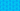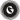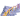PricingResources

# Cost plus pricing explainedWritten by

Cost-plus pricing is a way for businesses to determine the price they charge for a product or service. Sometimes referred to as markup pricing, it involves evaluating the cost of a product to the business, and then adding a percentage on top of that cost to create the price a customer can buy it for.

That additional percentage is the profit the company makes when it sells the product, thus the calculations involved in determining a cost-plus pricing strategy must be accurate.

While cost-plus pricing is a pretty simple pricing strategy, there are usually a variety of factors involved in determining the cost of a product or service to a business. For example, most products have a material cost, as well as labour and transport costs. All overheads involved in procuring materials, manufacturing, packaging, transporting and presenting the product to the customer must be considered to ensure a profitable markup is calculated. Also bear in mind that some overhead costs cannot be directly traced back to a specific source.

Let’s look closer at the process of a cost-plus pricing strategy.

## The cost-plus pricing process

The basic process involved in a cost-plus pricing strategy can be broken down into four stages. These stages include:

• determining the total cost

• dividing the total cost

• assigning a markup percentage

• multiplying the unit cost

Determine the total cost of the product or service. This is the sum of either fixed or variable costs, depending on the type of product or service in question, plus all overheads involved in the procurement, manufacture, labour and transport of the product.

Fixed costs do not vary, regardless of the number of units involved, and are thus more straightforward to calculate. Variable costs can vary according to different factors, such as a supplier discount achieved when a certain threshold amount is ordered. This could be a significant discount offered by the supplier when a certain number of units is ordered.

### Dividing the total cost

After determining the total cost of the product, divide the total cost by the number of units, which then gives you the cost of each individual unit. If your total cost was £1,000 for 100 units, then your unit cost will be £10.

### Assigning a markup percentage

This is the most complicated stage, as there are many variables at play which determine what percentage you choose to mark up a product. The markup is the percentage difference between the unit cost and the final selling price of the product, so this determines how much profit you make from each sale.

Estimate a competitor’s product markup from its unit cost and the price they are selling it for. Simply subtract the unit cost from the sales price and divide the resulting number by the unit cost. Then multiply the result by 100 to get the product’s markup percentage.

Determining the markup percentage for a brand new product is influenced by a variety of factors. For example, a lower markup means a lower price that could result in more sales if it is the sort of product that customers expect to be affordable.

Higher-end products may benefit from a higher markup percentage, and not just because you make more profit from each sale. A higher price signals luxury and thus could be attractive to customers who prefer shopping for higher-end goods.

### Multiplying the unit cost

The final stage involves multiplying the unit cost by the assigned markup percentage which gives you the selling price and the profit margin of each unit sold.

GoCardless is a global payments solution that helps you automate payment collection, cutting down on the amount of financial admin your team needs to deal with. Find out how GoCardless can help you with one-off or recurring payments.Contact sales

Sales

Support